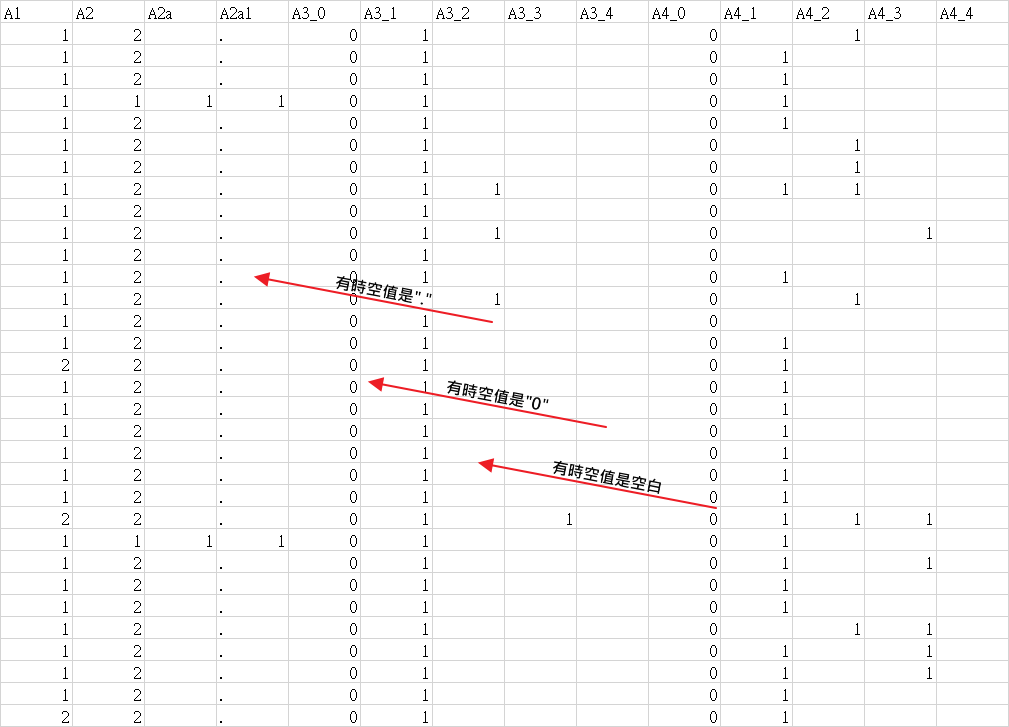#2019 iT 邦幫忙鐵人賽DAY 2
1
AI & Data

## [Day 2] 政府開放資料_計程車營運狀況調查(資處篇-1)``````#IF沒有請先安裝
library(tidyverse)
library(magrittr)
#x是資料本身
#y是變數名稱
#這一行在做快速分析的時候很好用，複製你要的資料然後跑這行資料就會讀進R_code，然後我對資料集x(資料本身)事先轉置了

#把NA值先全部改成-1
x[is.na(x)] = -1 #改成0的話會跟其他的搞混
for (i  in 1:149){  #共149個變數
#要把資料本身NA,0,1,2改成變數名稱，方便閱讀,用excel_vlookup的方式
for_vlookup = y[-(1:2),(i+1)]
for_vlookup = for_vlookup[-which(for_vlookup == "" )] %>%　as.character()#擷取一整行然後扣掉空值
a = 1:length(for_vlookup) #告訴我總共有多少變數
#把每一行都轉換成一個VLOOKUP用的表格
df1  = data.frame(
x1 = c(as.numeric(as.character(for_vlookup[a[a%%2==1]])),-1),
x2 = c(as.character(for_vlookup[a[a%%2==0]]),"miss值")
)
#每一行的資料
df2  = data.frame(
x1 =as.numeric(as.character(x[,i]))
)
#VLOOKUP
w =  df2 %>% inner_join(df1 , by = "x1")
#取代掉原本的數字,變成方便閱讀的中文
x[,i] = w[,2]
}
``````

df1是把資料從excel一行轉換成一個表格### 1 則留言

0

code解釋的好清楚喔XDDyanchen iT邦新手 5 級‧ 2018-10-19 22:06:44 檢舉